Скачать презентацию Results of serial dilution No lysis discrete plaques

ca4db74ae270a36c11f49b092251207e.ppt

• Количество слайдов: 13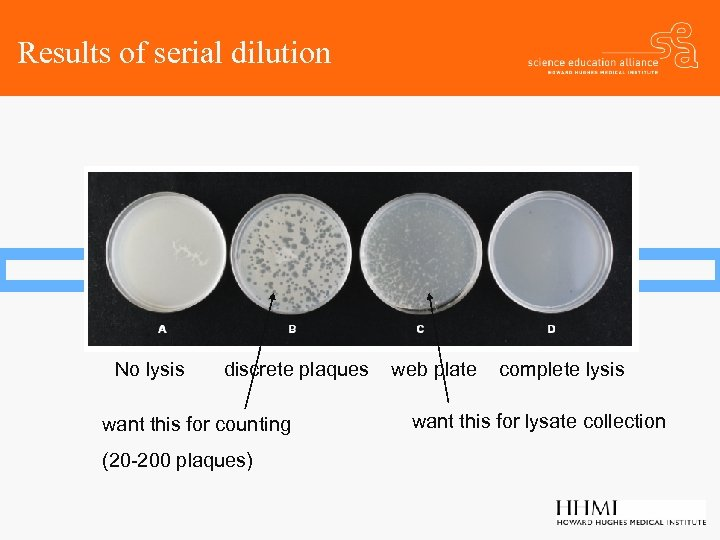Results of serial dilution No lysis discrete plaques want this for counting (20 -200 plaques) web plate complete lysis want this for lysate collection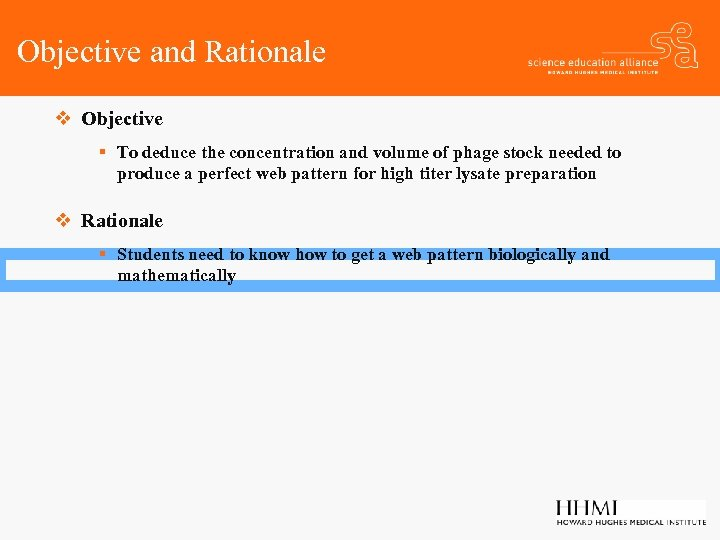Objective and Rationale v Objective § To deduce the concentration and volume of phage stock needed to produce a perfect web pattern for high titer lysate preparation v Rationale § Students need to know how to get a web pattern biologically and mathematically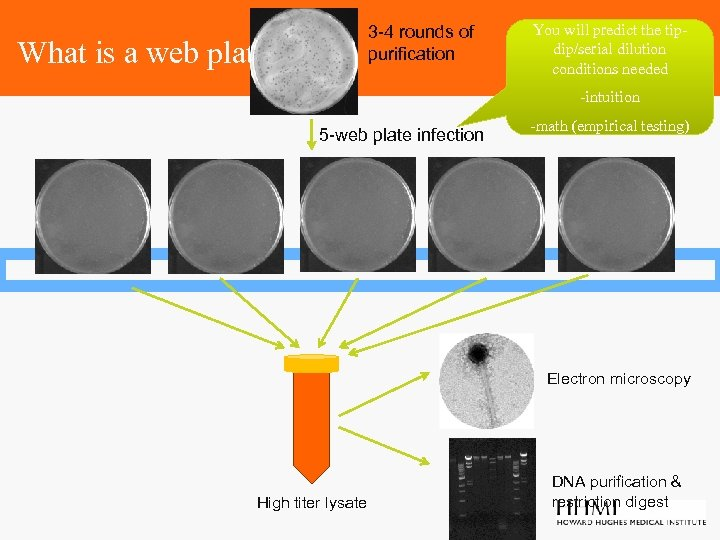3 -4 rounds of purification What is a web plate? You will predict the tipdip/serial dilution conditions needed -intuition 5 -web plate infection -math (empirical testing) Electron microscopy High titer lysate DNA purification & restriction digest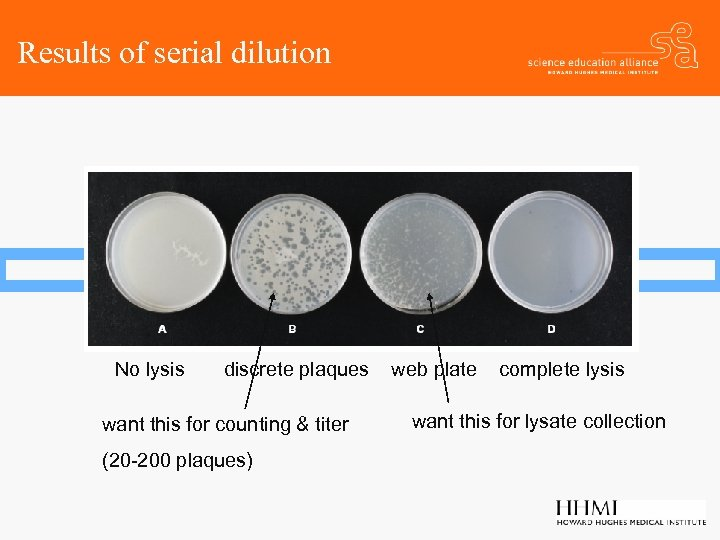Results of serial dilution No lysis discrete plaques want this for counting & titer (20 -200 plaques) web plate complete lysis want this for lysate collection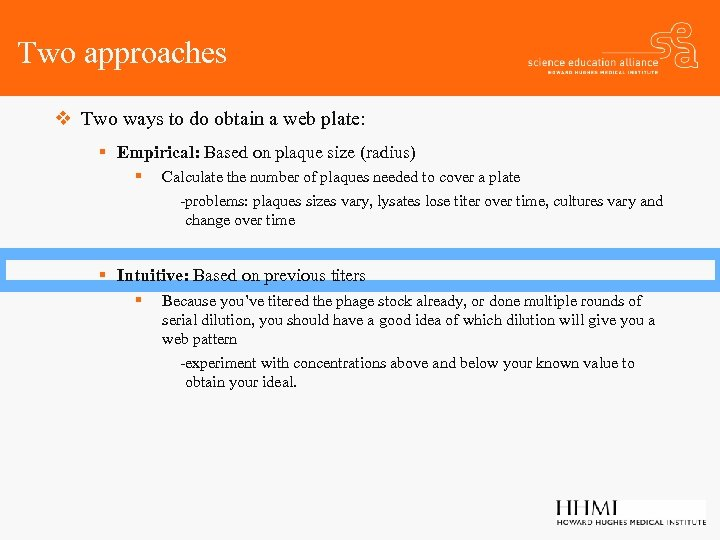Two approaches v Two ways to do obtain a web plate: § Empirical: Based on plaque size (radius) § Calculate the number of plaques needed to cover a plate -problems: plaques sizes vary, lysates lose titer over time, cultures vary and change over time § Intuitive: Based on previous titers § Because you’ve titered the phage stock already, or done multiple rounds of serial dilution, you should have a good idea of which dilution will give you a web pattern -experiment with concentrations above and below your known value to obtain your ideal.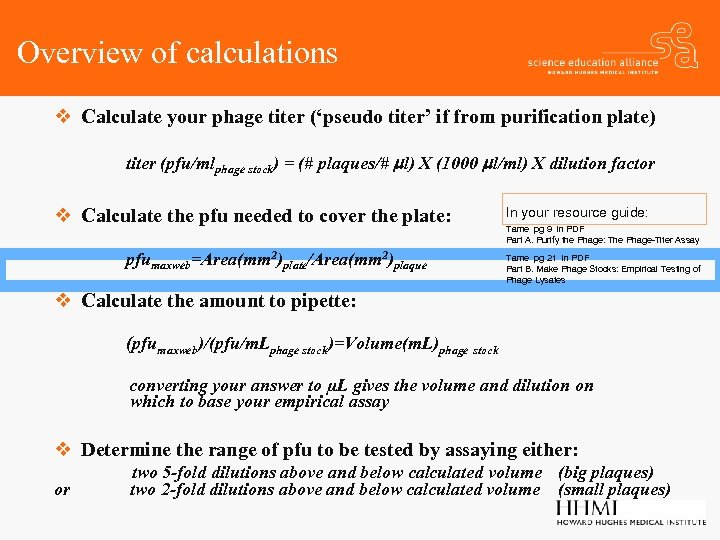Overview of calculations v Calculate your phage titer (‘pseudo titer’ if from purification plate) titer (pfu/mlphage stock) = (# plaques/# ml) X (1000 ml/ml) X dilution factor v Calculate the pfu needed to cover the plate: pfumaxweb=Area(mm 2)plate/Area(mm 2)plaque In your resource guide: Tame pg 9 in PDF Part A. Purify the Phage: The Phage-Titer Assay Tame pg 21 in PDF Part B. Make Phage Stocks: Empirical Testing of Phage Lysates v Calculate the amount to pipette: (pfumaxweb)/(pfu/m. Lphage stock)=Volume(m. L)phage stock converting your answer to µL gives the volume and dilution on which to base your empirical assay v Determine the range of pfu to be tested by assaying either: or two 5 -fold dilutions above and below calculated volume (big plaques) two 2 -fold dilutions above and below calculated volume (small plaques)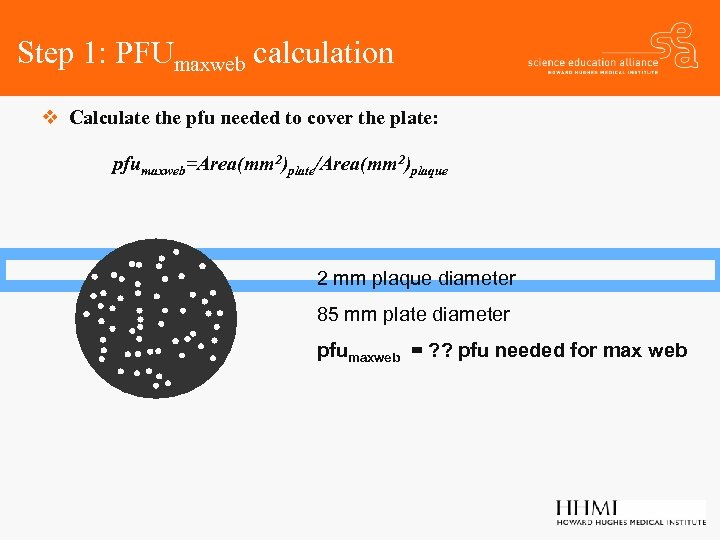Step 1: PFUmaxweb calculation v Calculate the pfu needed to cover the plate: pfumaxweb=Area(mm 2)plate/Area(mm 2)plaque 2 mm plaque diameter 85 mm plate diameter pfumaxweb = ? ? pfu needed for max web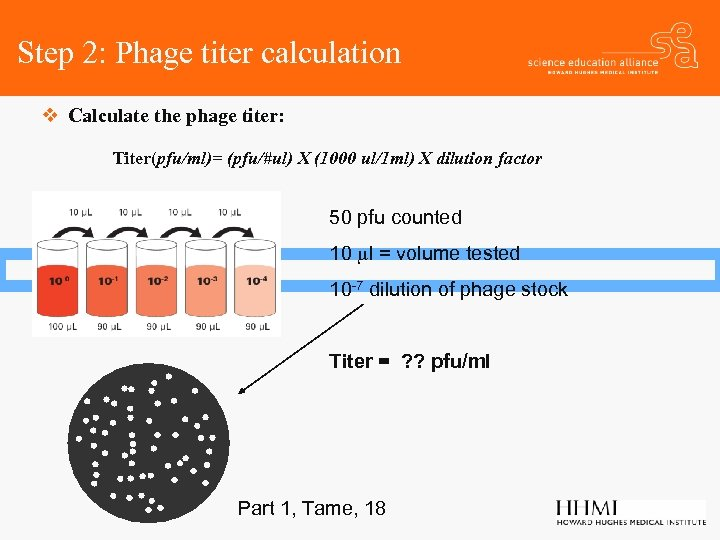Step 2: Phage titer calculation v Calculate the phage titer: Titer(pfu/ml)= (pfu/#ul) X (1000 ul/1 ml) X dilution factor 50 pfu counted 10 ml = volume tested 10 -7 dilution of phage stock Titer = ? ? pfu/ml Part 1, Tame, 18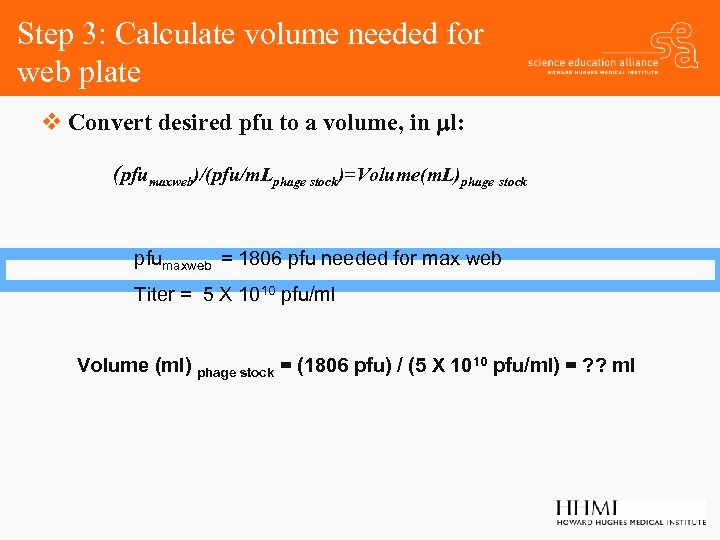Step 3: Calculate volume needed for web plate v Convert desired pfu to a volume, in ml: (pfumaxweb)/(pfu/m. Lphage stock)=Volume(m. L)phage stock pfumaxweb = 1806 pfu needed for max web Titer = 5 X 1010 pfu/ml Volume (ml) phage stock = (1806 pfu) / (5 X 1010 pfu/ml) = ? ? ml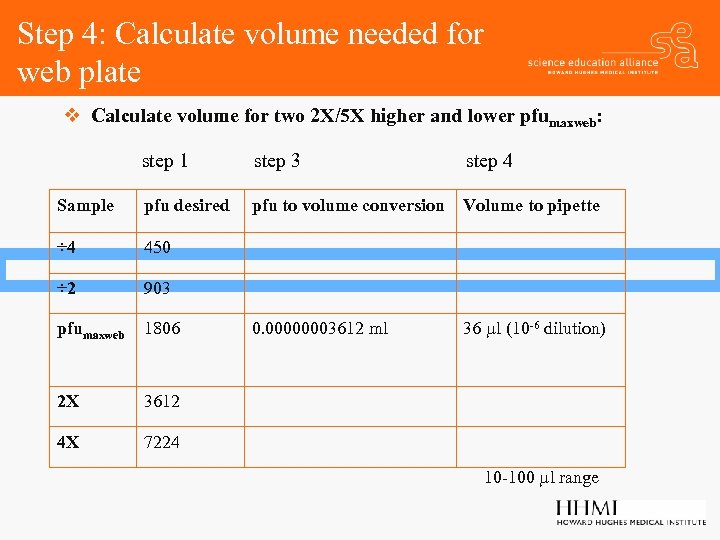Step 4: Calculate volume needed for web plate v Calculate volume for two 2 X/5 X higher and lower pfumaxweb: step 1 step 3 step 4 Sample pfu desired pfu to volume conversion Volume to pipette ÷ 4 450 ÷ 2 903 pfumaxweb 1806 0. 00000003612 ml 36 ml (10 -6 dilution) 2 X 3612 4 X 7224 10 -100 ml range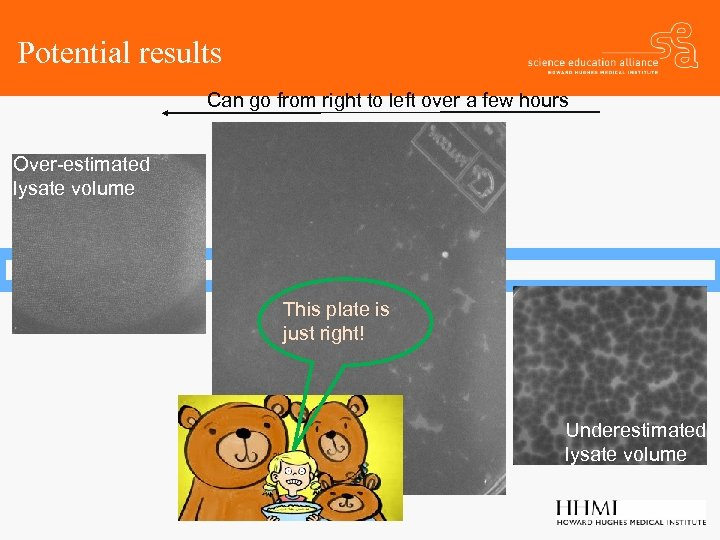Potential results Can go from right to left over a few hours Over-estimated lysate volume This plate is just right! Underestimated lysate volume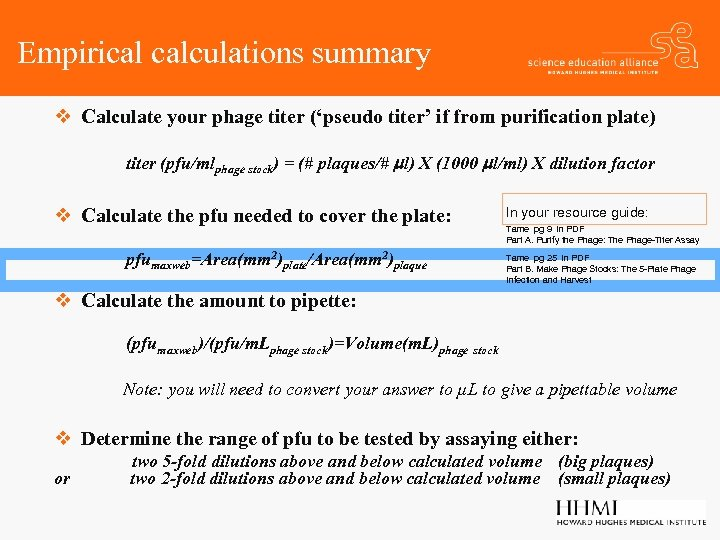Empirical calculations summary v Calculate your phage titer (‘pseudo titer’ if from purification plate) titer (pfu/mlphage stock) = (# plaques/# ml) X (1000 ml/ml) X dilution factor v Calculate the pfu needed to cover the plate: pfumaxweb=Area(mm 2)plate/Area(mm 2)plaque In your resource guide: Tame pg 9 in PDF Part A. Purify the Phage: The Phage-Titer Assay Tame pg 25 in PDF Part B. Make Phage Stocks: The 5 -Plate Phage Infection and Harvest v Calculate the amount to pipette: (pfumaxweb)/(pfu/m. Lphage stock)=Volume(m. L)phage stock Note: you will need to convert your answer to µL to give a pipettable volume v Determine the range of pfu to be tested by assaying either: or two 5 -fold dilutions above and below calculated volume (big plaques) two 2 -fold dilutions above and below calculated volume (small plaques)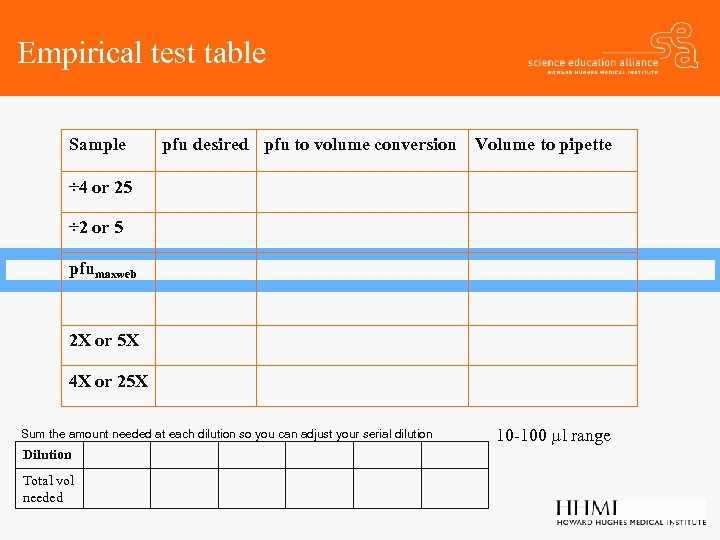Empirical test table Sample pfu desired pfu to volume conversion Volume to pipette ÷ 4 or 25 ÷ 2 or 5 pfumaxweb 2 X or 5 X 4 X or 25 X Sum the amount needed at each dilution so you can adjust your serial dilution Dilution Total vol needed 10 -100 ml range